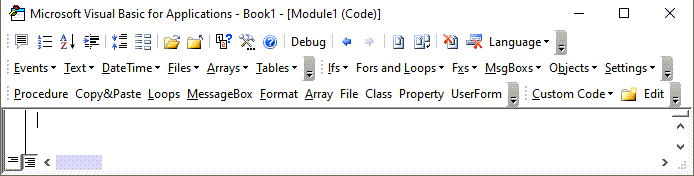# Delete or Insert Rows Based on Cell Value

This tutorial will demonstrate how to delete or insert rows based on cell values.

## Delete Row Based on Cell Value

This will loop through a range, and delete rows if column A says “delete”.

``````Sub DeleteRowsBasedonCellValue()

'Declare Variables
Dim LastRow As Long, FirstRow As Long
Dim Row As Long

With ActiveSheet
'Define First and Last Rows
FirstRow = 1
LastRow = .UsedRange.Rows(.UsedRange.Rows.Count).Row

'Loop Through Rows (Bottom to Top)
For Row = LastRow To FirstRow Step -1
If .Range("A" & Row).Value = "delete" Then
.Range("A" & Row).EntireRow.Delete
End If
Next Row
End With

End Sub``````

We must start the loop with the bottom row because deleting a row will shift the data, skipping rows if you loop top to bottom.

Also, notice that instead of manually entering in the last row, we calculate the last used row.

### Delete Row – Based on Filter

In the previous example, we looped through the rows, deleting each row that meets the criteria. Alternatively, we can use Excel’s AutoFilter to filter rows based on some criteria and then delete the visible rows:

``````Sub FilterAndDeleteRows()

'Declare ws variable
Dim ws As Worksheet
Set ws = ActiveSheet

'Reset Existing Filters
On Error Resume Next
ws.ShowAllData
On Error GoTo 0

'Apply Filter
ws.Range("a1:d100").AutoFilter Field:=1, Criteria1:="delete"

'Delete Rows
ws.Range("a1:d100").SpecialCells(xlCellTypeVisible).Delete

'Clear Filter
On Error Resume Next
ws.ShowAllData
On Error GoTo 0

End Sub``````

### Delete Row Based on Cell Criteria

This will loop through a range, deleting rows if the cell in column A meets certain criteria (< 0):

``````Sub DeleteRowsBasedonCellValue()

'Declare Variables
Dim LastRow As Long, FirstRow As Long
Dim Row As Long

With ActiveSheet
'Define First and Last Rows
FirstRow = 1
LastRow = .UsedRange.Rows(.UsedRange.Rows.Count).Row

'Loop Through Rows (Bottom to Top)
For Row = LastRow To FirstRow Step -1
If .Range("A" & Row).Value < 0 Then
.Range("A" & Row).EntireRow.Delete
End If
Next Row
End With

End Sub``````

### Delete Row if Cell is Blank

This will loop through a range, deleting a row if a cell in column A is blank:

``````Sub DeleteRowsBasedonCellValue()

'Declare Variables
Dim LastRow As Long, FirstRow As Long
Dim Row As Long

With ActiveSheet
'Define First and Last Rows
FirstRow = 1
LastRow = .UsedRange.Rows(.UsedRange.Rows.Count).Row

'Loop Through Rows (Bottom to Top)
For Row = LastRow To FirstRow Step -1
If .Range("A" & Row).Value = "" Then
.Range("A" & Row).EntireRow.Delete
End If
Next Row
End With

End Sub``````

### Delete Blank Row

Alternatively, if you want to delete a row if the entire row is blank (Click link for a slightly different method), you can use this code:

``````Sub DeleteBlankRows()

'Declare Variables
Dim LastRow As Long, FirstRow As Long
Dim Row As Long

With ActiveSheet
'Define First and Last Rows
FirstRow = 1
LastRow = .UsedRange.Rows(.UsedRange.Rows.Count).Row

'Loop Through Rows (Bottom to Top)
For Row = LastRow To FirstRow Step -1
If WorksheetFunction.CountA(.Rows(Row)) = 0 Then
.Rows(Row).EntireRow.Delete
End If
Next Row
End With

End Sub``````

### Delete Row if Cell Contains Value

This will loop through a range, deleting a row if the cell in column A is not blank:

``````Sub DeleteRowsBasedonCellValue()

'Declare Variables
Dim LastRow As Long, FirstRow As Long
Dim Row As Long

With ActiveSheet
'Define First and Last Rows
FirstRow = 1
LastRow = .UsedRange.Rows(.UsedRange.Rows.Count).Row

'Loop Through Rows (Bottom to Top)
For Row = LastRow To FirstRow Step -1
If .Range("A" & Row).Value <>"" Then
.Range("A" & Row).EntireRow.Delete
End If
Next Row
End With

End Sub``````

## VBA Coding Made Easy

Stop searching for VBA code online. Learn more about AutoMacro - A VBA Code Builder that allows beginners to code procedures from scratch with minimal coding knowledge and with many time-saving features for all users!## Insert Row Based on Cell Value

This will loop through a range, inserting rows if a certain cell in that row says “insert”:

``````Sub InsertRowsBasedonCellValue()

'Declare Variables
Dim LastRow As Long, FirstRow As Long
Dim Row As Long

With ActiveSheet
'Define First and Last Rows
FirstRow = 1
LastRow = .UsedRange.Rows(.UsedRange.Rows.Count).Row

'Loop Through Rows (Bottom to Top)
For Row = LastRow To FirstRow Step -1
If .Range("A" & Row).Value = "insert" Then
.Range("A" & Row).EntireRow.Insert
End If
Next Row
End With

End Sub``````